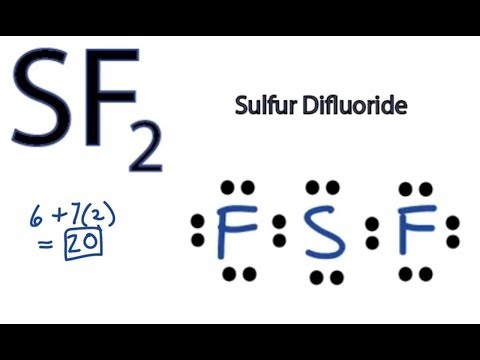Resources:

Have you found videos, websites, or explanations that helped you understand this chapter? Let us know and we'll add them to "Resources" part of this page for other students to use.

Question 1

Predict the hybridization of the left carbon atom inSelect one:Explanation

C: 4 bonds  + 0 lone pairs = 4 electron groupQuestion 2

Predict the hybridization of the right carbon atom inSelect one:Explanation

C : 3 bonds + 0 lone pairs = 3 electron groupQuestion 3

Predict the hybridization of the oxygen atom inSelect one:Explanation

O : 1 bond + 2 lone = 3 electron groupQuestion 4

Predict the hybridization of the carbon atoms inSelect one:Explanation

C : 4 bonds + 0 lone pair = 4 electron groupQuestion 5

Predict the hybridization of the oxygen atom inSelect one:Explanation

O : 2 bonds + 2 lone pairs = 4Question 6

Aspirin, or acetylsalicylic acid, has the formula C9H8O4 and the skeleton structure shown below. In order to answer the question first Complete the Lewis structure. What is the number of sigma bonds in aspirin?ExplanationQuestion 7

What is the number of pi bonds in aspirin?ExplanationQuestion 8

What is the hybridization about the COOH carbon atom (colored blue)?

Select one:ExplanationC : 3 bonds + 0 lone = 3Question 9

What is the hybridization about the carbon atom in the benzene-like ring that is bonded to an oxygen atom (colored magenta)?

Select one:Explanation

C: 3 bonds + 0 lone pairs = 3

trigonal planarQuestion 10

Also, what is the hybridization of the oxygen atom bonded to this carbon atom?

Select one:Explanation

O : 1 bond + 2 lone pair = 3Question 11

Use the molecular orbital diagrams in the course notes to predict which species in each pair has the stronger bond.

Select one or more:Explanation

The element with a negative charge has more electrons than protons. The more electrons the stronger the charge.

Rule of Thumb: The element with more electrons has a stronger bond.

Question 12

The molecular orbital diagram CO in the course notes also applies to the following species. Determine the bond order of LiBe+.Explanation

Bond order = (bonding−anti-bonding) / 2

2 electrons bonded /2 = 1

Question 13

How many unpaired electrons are in LiBe+ and is it paramagnetic?

Select one or more:Explanation

Both Li and Be+ have complete electron configurations

Question 14

The molecular orbital diagram CO in the course notes to determine the bond order of CO+.Explanation

Bond order = (bonding−anti-bonding) / 2

(3 electrons bonded + 2 free electons)/2 = 2.5

Question 15

CO+ has how many unpaired electrons and is is paramagnetic?

Select one or more:ExplanationQuestion 16

The molecular orbital diagram CO in the course notes also applies to the following species. Determine the bond order of CN-.Explanation

Bond order = [# of bonding electrons -  # of antibonding electrons]/2

= [8-2]/2 =3

Question 17

CN- has how many unpaired electrons and is is paramagnetic?

Select one:ExplanationQuestion 18

The molecular orbital diagram CO in the course notes also applies to the following species. Determine the bond order of OF.Explanation

Bond order = [No of bonding electrons- No of antibonding electrons]/2

= [4 - 1]/2 = 1.5

Question 19

OF has how many unpaired electrons and is it paramagnetic?

Select one or more:Explanation

OF has 1 unpaired electron and is paramagnetic

Question 20

Formamide, HC(O)NH2, is prepared at high pressures from carbon monoxide and ammonia and serves as an industrial solvent.

H-C-N-H (showing how atoms are bonded)
|    |
O H
Two resonance forms can be written for formamide which satisfy the octet rule (one with no formal charges and one with formal charges). Write both resonance structures. For the resonance structure with no formal charges, what is the O-C-N bond angle ?

Select one:Explanation

O-C-N : 3 bonds + 0 lone pairs = Hybridization: sp2Question 21

For the resonance structure with no formal charges, what is the C-N-H bond angle?

Select one:Explanation

C-N-H : 3 bonds + 1 lone : Hybridization: sp3Question 22

For the resonance structure with formal charges, what is the O-C-N bond angle ?

Select one:Explanation

C: 3 bonds + 0 lone pairs = Hybridization: sp2

Question 23

For the resonance structure with formal charges, what is the C-N-H bond angle ?

Select one:Explanation

N: 3 bonds + 0 lone pair = Hybridization sp2

Question 24

Is it possible to use experimental bond angles to determine which resonance structure is more important?

Select one:Explanation

The resonance structure that minimize the charge to 0 which corresponds to the charge of the formula HC(O)NH2

Question 25

The reaction of sulfur, S8, with fluorine, F2, yields a product with the general formula SFx. If 4.01 g of S8 react with 4.76 g of F2 to yield only SFx, what is the value of x?Explanation

256g= 1 mole of S8
4.01g= 4.01/256
= 0.0157 moles of S8

38g= 1 mole of F2
4.76g= 4.76/38
= 0.125 moles of F2

1 mole of S8=" 8" moles of S atoms
0.0157 moles of S8=" (8)(0.0157)
= 0.1256 moles of S atoms(this is equal to the no of moles of S atoms on the right)

1 mole of F2=" 2" moles of F atoms
0.125 moles of F2=" 2(0.125)
= 0.2500 moles of F atoms(this is equal to the no of moles of F atoms on the right)

The ratio of the no of S atoms to F atoms in the product is ( S:F) 0.1256:0.2500= 1:2.
Therefore, x=" 2

Question 26

Draw the Lewis structure of this compound, indicating the F-S-F bond angles and the hybrid orbitals used by sulfur.

Select one:ExplanationS: 2 bonds + 2 lone pairs= 4 tetrahedral  sp3.